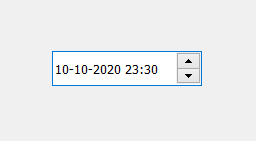Skip to content
Related Articles
PyQt5 – QDateTimeEdit
• Last Updated : 10 Jul, 2020

QDateTimeEdit allows the user to edit dates by using the keyboard or the arrow keys to increase and decrease date and time values. The arrow keys can be used to move from section to section within the QDateTimeEdit box. Dates and times appear in accordance with the format set. Below is how the QDateTimeEdit looks likeExample :
A window having a QDateTimeEdit widget and the label and every time the value in date-time widget changes labels will show the current date-time.

Below is the implementation

 `# importing libraries``from` `PyQt5.QtWidgets ``import` `*` `from` `PyQt5 ``import` `QtCore, QtGui``from` `PyQt5.QtGui ``import` `*` `from` `PyQt5.QtCore ``import` `*` `import` `sys`` ` ` ` `class` `Window(QMainWindow):`` ` `    ``def` `__init__(``self``):``        ``super``().__init__()`` ` `        ``# setting title``        ``self``.setWindowTitle(``"Python "``)`` ` `        ``# setting geometry``        ``self``.setGeometry(``100``, ``100``, ``500``, ``400``)`` ` `        ``# calling method``        ``self``.UiComponents()`` ` `        ``# showing all the widgets``        ``self``.show()`` ` `    ``# method for components``    ``def` `UiComponents(``self``):`` ` `        ``# creating a QDateTimeEdit widget``        ``datetime ``=` `QDateTimeEdit(``self``)`` ` `        ``# setting geometry``        ``datetime.setGeometry(``100``, ``100``, ``150``, ``35``)`` ` `        ``# creating a label``        ``label ``=` `QLabel(``"GeeksforGeeks"``, ``self``)`` ` `        ``# setting geometry to the label``        ``label.setGeometry(``100``, ``160``, ``200``, ``60``)`` ` `        ``# making label multi line``        ``label.setWordWrap(``True``)`` ` `        ``# adding action to the datetime``        ``datetime.dateTimeChanged.connect(``lambda``: dt_method())`` ` `        ``# method called by the datetime``        ``def` `dt_method():`` ` `            ``# getting current datetime``            ``value ``=` `datetime.dateTime()`` ` `            ``# setting text to the label``            ``label.setText(``"Current date time : "` `+` `str``(value))`` ` ` ` ` ` ` ` `# create pyqt5 app``App ``=` `QApplication(sys.argv)`` ` `# create the instance of our Window``window ``=` `Window()`` ` `# start the app``sys.exit(App.``exec``())`

Output :

Attention geek! Strengthen your foundations with the Python Programming Foundation Course and learn the basics.

To begin with, your interview preparations Enhance your Data Structures concepts with the Python DS Course. And to begin with your Machine Learning Journey, join the Machine Learning – Basic Level Course

My Personal Notes arrow_drop_up